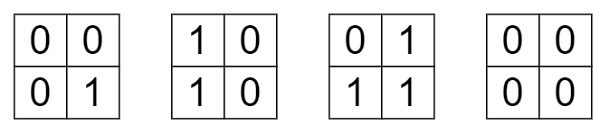# Minimum Number of Flips to Convert Binary Matrix to Zero Matrix in C++

Suppose we have a m x n binary matrix mat. In one step, we can choose one cell and flip its bit and all the four neighbors of it if they are present. We have to find the minimum number of steps required to convert mat to a zero matrix. If there is no solution, then return -1.

So if the given input is like [[0,0], [0,1]], alteration will be like −So we need 3 steps, the output will be 3.

To solve this, we will follow these steps −

• n := number of rows, m := number of columns, x := 0
• for initialize i := 0, when i < n, update (increase i by 1), do −
• for initialize j := 0, when j < m, update (increase j by 1), do −
• for initialize j := 0, when j < m, update (increase j by 1), do −
• x := x + left shift mat[i][j] value (i * m) + j) number of times
• Define an array dp with 2^(n * m) cells, fill this with -1
• dp[x] := 0
• Define one queue q
• insert x into q
• while (q is not empty), do −
• current := first element of q, delete element from q
• if current is same as 0, then −
• return dp[current]
• for initialize i := 0, when i < n, update (increase i by 1), do −
• for initialize j := 0, when j < m, update (increase j by 1), do −
• temp := current
• temp := temp XOR 2^ (i * m) + j)
• for initialize k := 0, when k < 4, update (increase k by 1), do −
• ni := i + dir[k]
• nj := j + dir[k]
• if ni < 0 or nj < 0 or ni >= n or nj >= m, then −
• temp := temp XOR 2^ (ni * m) + nj)
• if dp[temp] is same as -1, then −
• dp[temp] := dp[current] + 1
• insert temp into q
• return -1

Let us see the following implementation to get better understanding −

## Example

Live Demo

#include <bits/stdc++.h>
using namespace std;
int dir = {{1, 0}, {-1, 0}, {0, -1}, {0, 1}};
class Solution {
public:
int minFlips(vector<vector<int>>& mat) {
int n = mat.size();
int m = mat.size();
int x = 0;
for(int i = 0; i < n; i++){
for(int j = 0; j < m; j++){
x += (mat[i][j] << ((i * m) + j));
}
}
vector < int > dp(1 << (n*m), -1);
dp[x] = 0;
queue <int> q;
q.push(x);
while(!q.empty()){
int current = q.front();
q.pop();
if(current == 0)return dp[current];
for(int i = 0; i < n; i++){
for(int j = 0; j < m; j++){
int temp = current;
temp ^= (1 << ((i *m) + j));
for(int k = 0; k < 4; k++){
int ni = i + dir[k];
int nj = j + dir[k];
if(ni < 0 || nj < 0 || ni >= n || nj >= m)continue;
temp ^= (1 << ((ni *m) + nj));
}
if(dp[temp] == -1){
dp[temp] = dp[current] + 1;
q.push(temp);
}
}
}
}
return -1;
}
};
main(){
Solution ob;
vector<vector<int>> v = {{0,0},{0,1}};
cout << (ob.minFlips(v));
}

## Input

{{0,0},{0,1}}

## Output

3